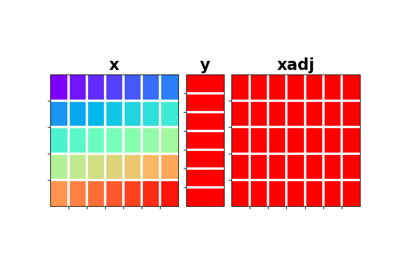# pylops.Sum¶

class pylops.Sum(dims, dir, dtype='float64')[source]

Sum operator.

Sum along an axis of a multi-dimensional array (at least 2 dimensions are required) in forward model, and spread along the same axis in adjoint mode.

Parameters: dims : tuple Number of samples for each dimension dir : int Direction along which summation is performed. dtype : str, optional Type of elements in input array.

Notes

Given a two dimensional array, the Sum operator re-arranges the input model into a multi-dimensional array of size dims and sums values along direction dir:

$y_j = \sum_i x_{i, j}$

In adjoint mode, the data is spread along the same direction:

$x_{i, j} = y_j \quad \forall i=0, N-1$
Attributes: shape : tuple Operator shape explicit : bool Operator contains a matrix that can be solved explicitly (True) or not (False)

Methods

 __init__(dims, dir[, dtype]) Initialize this LinearOperator. adjoint() Hermitian adjoint. apply_columns(cols) Apply subset of columns of operator cond([uselobpcg]) Condition number of linear operator. conj() Complex conjugate operator div(y[, niter]) Solve the linear problem $$\mathbf{y}=\mathbf{A}\mathbf{x}$$. dot(x) Matrix-matrix or matrix-vector multiplication. eigs([neigs, symmetric, niter, uselobpcg]) Most significant eigenvalues of linear operator. matmat(X) Matrix-matrix multiplication. matvec(x) Matrix-vector multiplication. rmatmat(X) Matrix-matrix multiplication. rmatvec(x) Adjoint matrix-vector multiplication. todense([backend]) Return dense matrix. toimag([forw, adj]) Imag operator toreal([forw, adj]) Real operator tosparse() Return sparse matrix. trace([neval, method, backend]) Trace of linear operator. transpose() Transpose this linear operator.

## Examples using pylops.Sum¶Sum